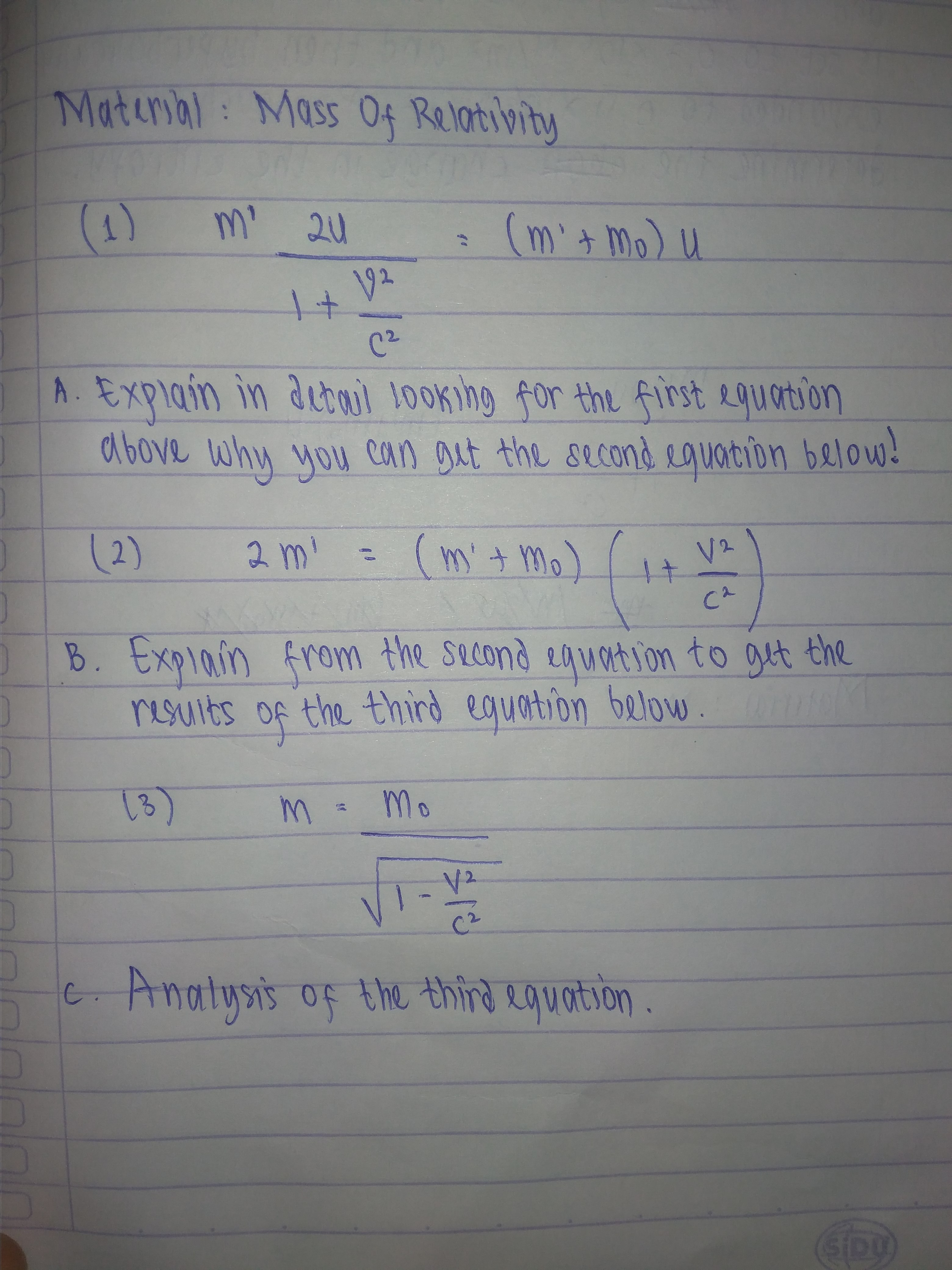# Maturyal Mass 04 Relativity(m mo) u20V2+C2A. Explain in aebul Jooking for the first aquartionabove why youCaul 100king for the firstROuartsoncan git the second cquotion below.2)2 mm' mV2CAB. ExpIain from the sucond enuartion to gtt theriSuits of the third equation below13)MomV2C2C Anatysis of the third equotion

Question
26 viewshelp_outlineImage TranscriptioncloseMaturyal Mass 04 Relativity (m mo) u 20 V2 + C2 A. Explain in aebul Jooking for the first aquartion above why you Caul 100king for the first ROuartson can git the second cquotion below . 2) 2 m m' m V2 CA B. ExpIain from the sucond enuartion to gtt the riSuits of the third equation below 13) Mo m V2 C2 C Anatysis of the third equotion fullscreen
check_circle

Step 1
1. A) Write the first equation (1) from the question.
Step 2

Multiply and divide both the sides by 1+v2/c2  and u respectively to get the required equation (2).

Step 3

B)

It can be easily checked when we substitute value of m’ from equation (3) ,the L.H.S and R.H.S is not equal this means that equation (3) cannot be derived by just rearranging the equation (2).

This can be only...

### Want to see the full answer?

See Solution

#### Want to see this answer and more?

Solutions are written by subject experts who are available 24/7. Questions are typically answered within 1 hour.*

See Solution
*Response times may vary by subject and question.
Tagged in

### Science